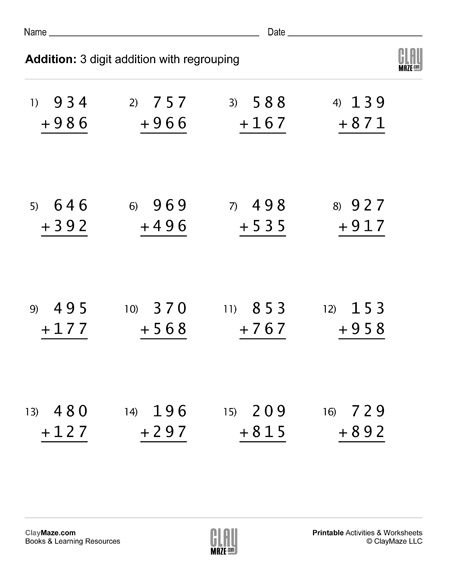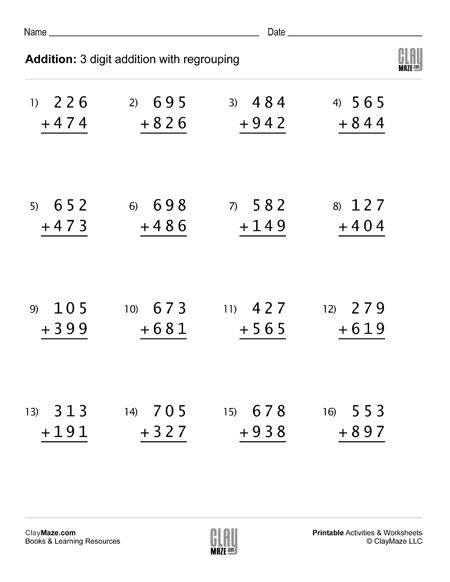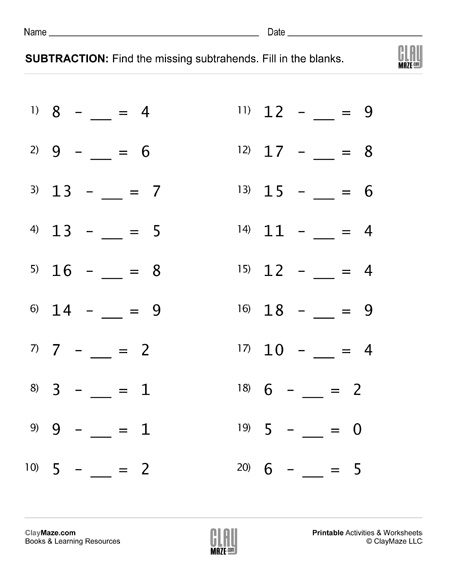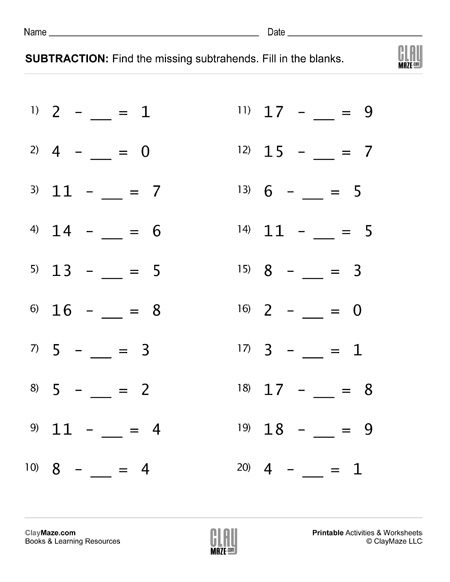Free Printable Worksheets for Second Grade

Even Numbers and Odd Numbers (Set 4)Identify the even numbers and odd numbers in this worksheet. Find the even numbers in the first section and then find the odd numbers in the second section. Great for first grade and second grade....

3 digit addition worksheet with regrouping (Set 1)3 digit addition worksheet with regrouping (Set 2)3 digit addition worksheet with regrouping (Set 3)This math worksheet contains 16 problems of 3 digit addition with regrouping – good for first grade, second and third grade addition practice....

3 digit addition worksheet with regrouping (Set 4)Free math addition worksheet with 3 digit numbers. All problems in this set are on addition with regrouping....

Build Your Own Math Problems – Fun Paper Cut OutsThis is a great rainy day activity sheet. This math learning project is a little more interactive in that the child gets to cut out the squares necessary to build the problems and then can either a...

Learn About Isograms – Word Building ActivityAn Isogram, otherwise known as a “nonpattern word”, is just a fancy way of referring to a word or phrase that has no repeating letters. This particular activity not only introduces the child to Iso...

Subtraction Worksheet – Fill in the Blanks Subtraction Facts (Set 1)Subtraction practice worksheet. Fill in the blanks – Find the missing subtrahend. Solutions included in download....

Subtraction Worksheet – Fill in the Blanks Subtraction Facts (Set 2)Fill in the blank subtraction worksheet. 20 problems. – with solutions...

Subtraction Worksheet – Fill in the Blanks Subtraction Facts (Set 3)Worksheet for practice with subtraction facts. Fill in the blanks. Solutions included....

Subtraction Worksheet – Fill in the Blanks Subtraction Facts (Set 4)Subtraction worksheet. Fill in the blanks with the correct subtrahends. Solutions are on page 2 of the pdf....